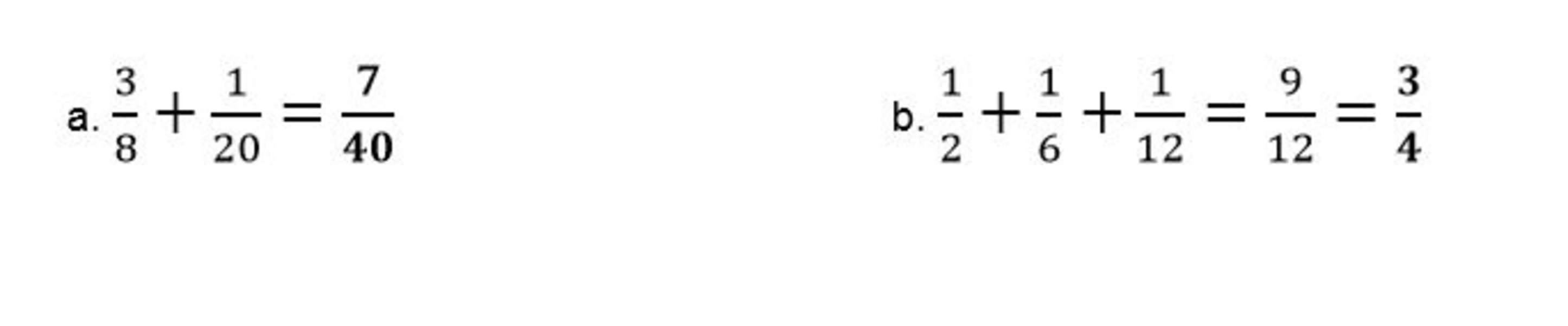## Step 1: Check the denominators are different

Confirm the denominators of the fractions are different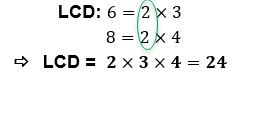## Step 2: Find the least common denominator

Next you need to find the least common denominator (LCD)## Step 3: Rewrite the fractions so they share the same denominator

Multiply the numerator with the factor needed to come up with the least common denominator.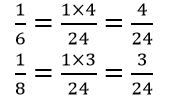## Step 4: Add the numerators

Since the denominator are now alike, add the numerators and write LCD as the denominator to find the sum.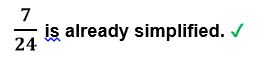## Step 5: Simplify fractions

Simplify the sum if needed.## Examples on adding fractions with different denominators

Q1) Find the sum of the following sets of fractions: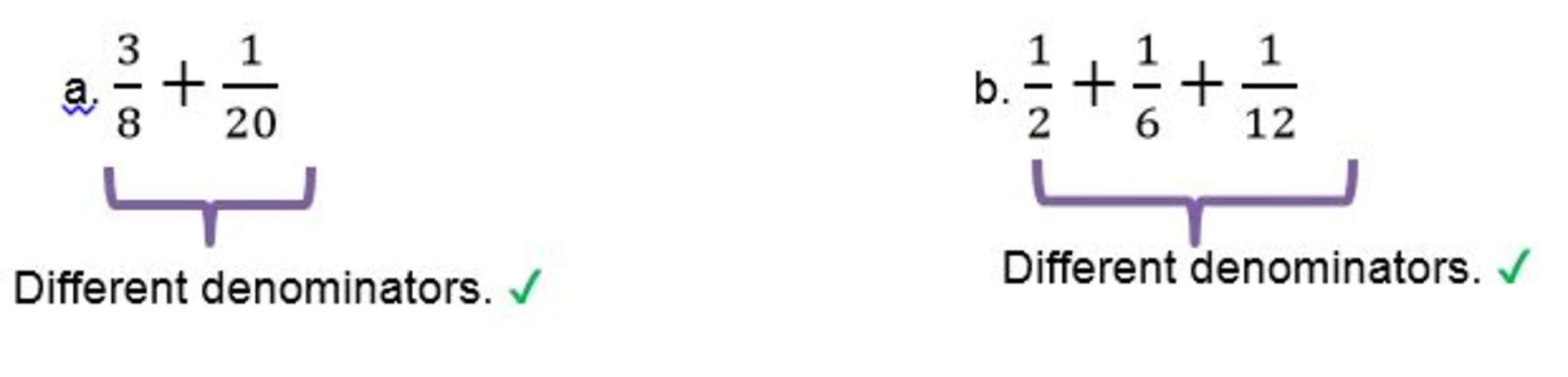Find the least common denominator shared by the sets of fractions.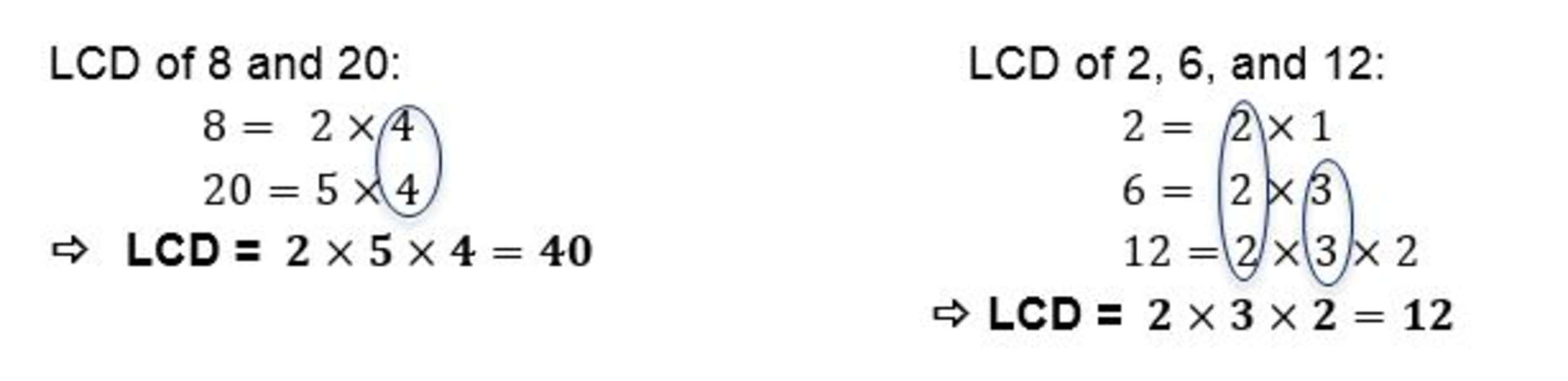Express the fractions so that they share common denominators.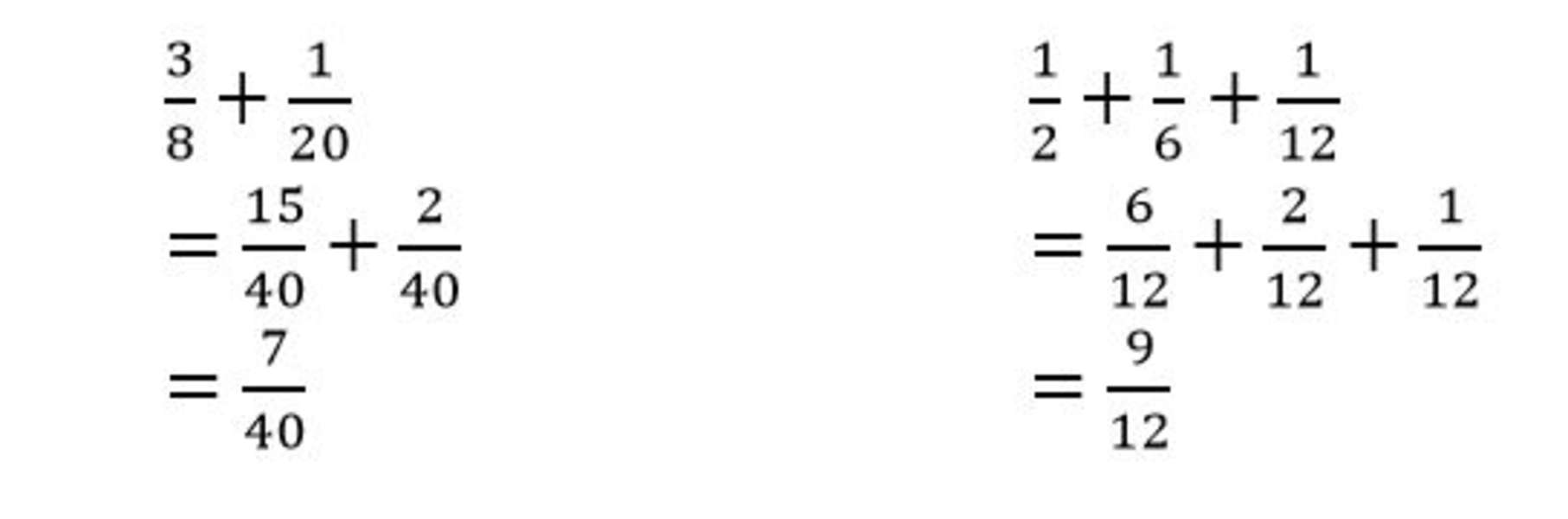Write the new fractions and find the sum by adding the numerators.Simplify the sum when needed.